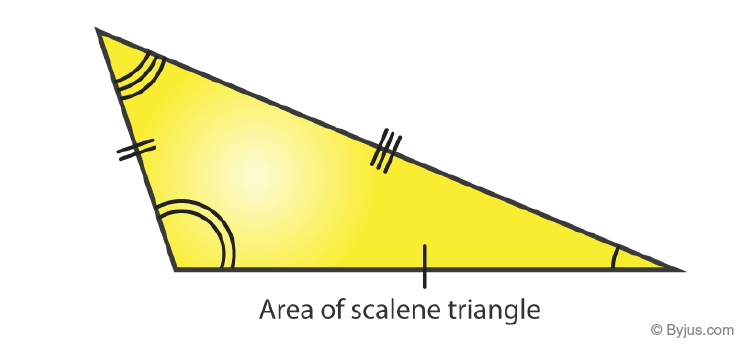# Area of Scalene Triangle

A Scalene triangle has three random(Unequal) sides/lengths and three random (unequal) angles.

A simple definition of a scalene triangle is “A Scalene triangle is a triangle with three different sides and angles.”

Example: The sail on a sailboat is also likely to be raised in the shape of a scalene triangle, with no side of the sail being the same length as any other side.As there are different types of triangles, how is the scalene triangle different from other triangles? (Read the Difference table below).

 Differences Scalene Isosceles Equilateral Sides 3 sides with random lengths 2 Equal Sides 3 Equal Sides Angles 3 random angles One Right Angle(90) 3 Equal Angles

## Area of Scalene Triangle Formula

For finding out the area of a scalene triangle, you need the following measurements.

a) The length of one side and the perpendicular distance of that side to the opposite angle.

b) The lengths of all three sides.

### Area of Scalene Triangle With Base and Height

The area of a scalene triangle with any side as base ‘b’ and height ‘h’ (an altitude on that base) is

$$\begin{array}{l}A = \frac{1}{2}\times b\times h square\ units\end{array}$$

### Area of Scalene Triangle Example

Q1) Find the area of the triangle with base 20 cm and height 12 cm?

Solution: Base (b) = 20 cm

Height (h) = 12cm

$$\begin{array}{l}A =\frac{1}{2}\times b\times h\end{array}$$
$$\begin{array}{l}= \frac{1}{2}\times 20\times 12\end{array}$$

=120cm2

### Area of Scalene Triangle Using Heron’s Formula

Step 1: If you know the length of the three sides of the triangle (a, b, c).

Step 2: Find the semi-perimeter, S.

The formula for finding the semi-perimeter of a triangle is

$$\begin{array}{l}S =\frac{a+b+c}{2}\end{array}$$

Step 3: Then apply the Heron’s formula to find the area of a scalene triangle

$$\begin{array}{l}A=\sqrt{S(S-a)(S-b)(S-c)} square\ units\end{array}$$

### Area of a Scalene Triangle with Three Sides

Q1) Find the area of a triangle whose sides are 122 cm, 120 cm, and 22 cm.

Solution:

$$\begin{array}{l}S =\frac{a+b+c}{2}\end{array}$$
$$\begin{array}{l} = \frac{122+120+22}{2} cm\end{array}$$
$$\begin{array}{l}= \frac{264}{2} cm\end{array}$$

S = 132 cm

$$\begin{array}{l}A =\sqrt{S(S-a)(S-b)(S-c)}\end{array}$$
$$\begin{array}{l}= \sqrt{132(132-122)(132-120)(132-22)} cm^{2}\end{array}$$
$$\begin{array}{l}=\sqrt{132(10)(12)(110)}cm^{2}\end{array}$$
$$\begin{array}{l} =\sqrt{12\times 11\times 10\times 12\times 11\times 10}cm^{2}\end{array}$$
$$\begin{array}{l}= \sqrt{12^{^{2}}\times 11^{2}\times 10^{2}}cm^{2}\end{array}$$
$$\begin{array}{l}=\sqrt{1320^{2}}cm^{2}\end{array}$$

Therefore, the area of a triangle is 1320 cm2

### Area of Scalene Triangle without Height

The value of one of the angles (Suppose ∠C) as well as the lengths of the two sides (a and b) that form a scalene triangle, measures the area as:

$$\begin{array}{l}Area = \frac{ab}{2}\times Sin C\end{array}$$

### Practice Example

Q1) Find the area of the triangle with two sides as 28 cm and 35 cm and the angle between these sides as 60°?

Solution:

$$\begin{array}{l}Area =\frac{ab}{2}\times Sin C\end{array}$$
$$\begin{array}{l}Area =\frac{28\times 35}{2}\times sin 60^{\circ} cm^{2}\end{array}$$
$$\begin{array}{l}=\frac{28\times 35}{2}\times \frac{\sqrt{3}}{2} cm^{2}\end{array}$$
$$\begin{array}{l}= 490 \frac{\sqrt{3}}{2} cm^{2}\end{array}$$

To practice more questions on various Maths topics, Download the BYJU’S app.

Quiz on Area of Scalene Triangle